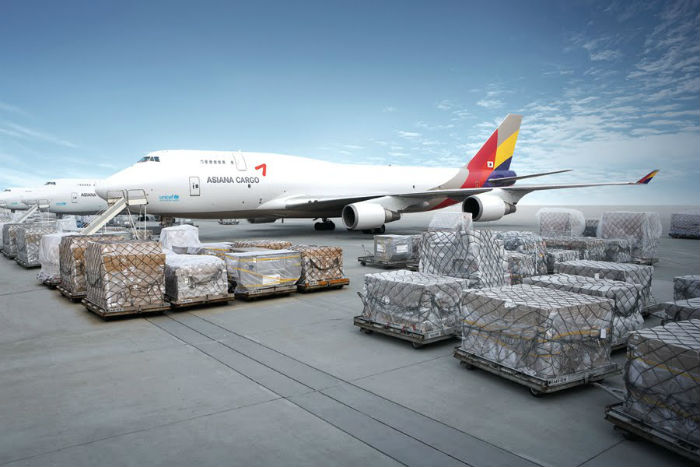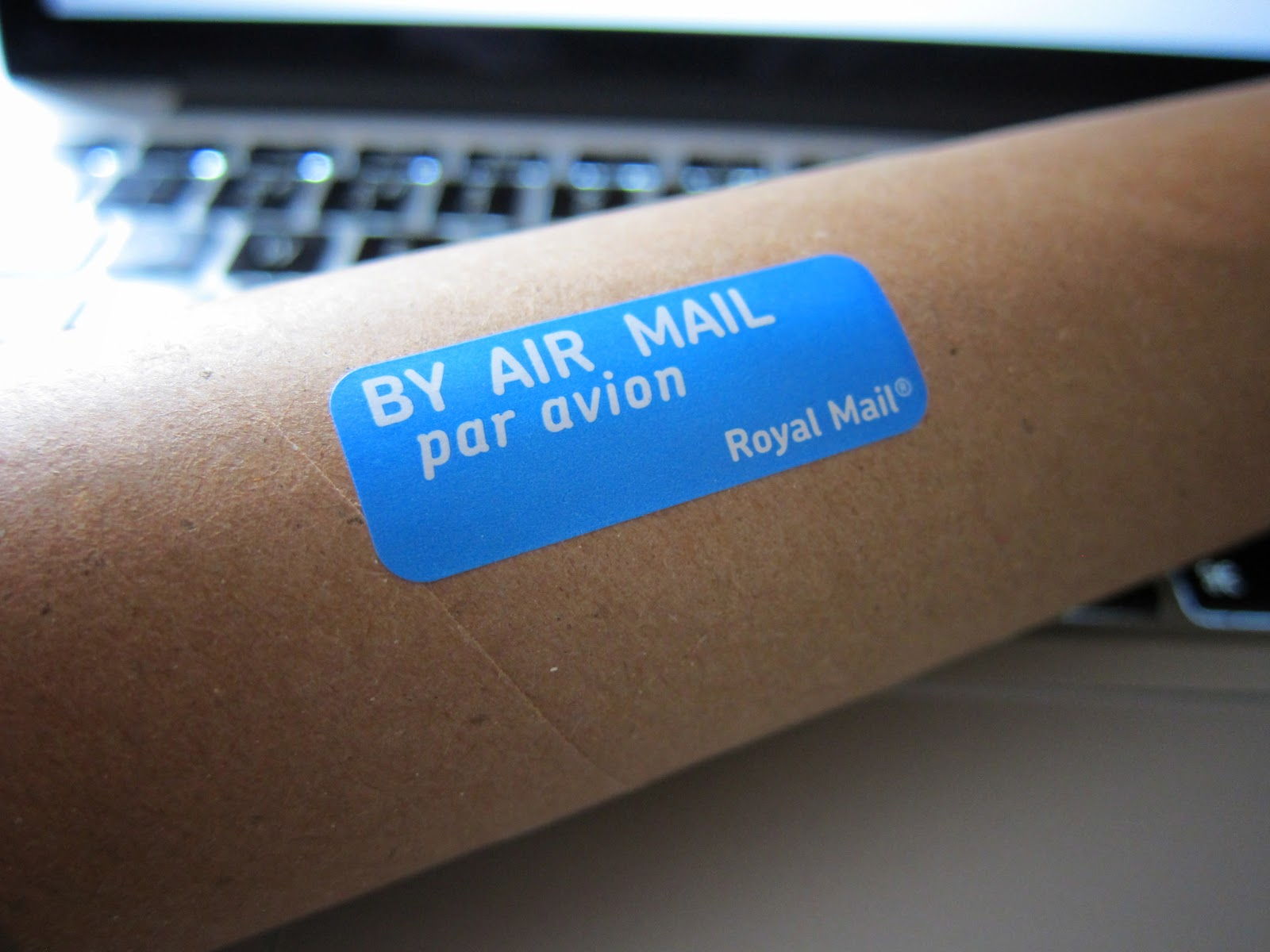## How to Calculate for The Air Freight Volumetric Weight?Air Freight companies use different dimensions and weights to calculate their transportation fees. If you’d like to get an understanding of how they do this, here is a rundown on the calculation of air freight volumetric weight, also called dimensional weight. You will also learn how transportation companies make out their fee using these variables and manage international business transactions successfully.## Gross weight

Amongst common methods adopted by transportation companies when accounting for air freight volume is finding out total weight. This unit of measurement accounts all packaging material, the weight of the contents of the entire parcel, as well as any cushioning to protect that parcel in transit. Conducting the air cargo load calculation depends on various variables, like the type of packaging used as well as the weight of the parcel.

If you choose to palletize your parcels, the pallets will be accounted for in the gross weight. Finding out the gross weight is essential in calculating the air freight volume.

To try and reduce the gross weight, consider less bulky and lighter packaging materials especially if you are sending later parcels. When doing this, do not compromise the safety and security of the airplane that will be carrying your parcels.

If the parcel requires specialized packaging due to safety and security purposes, comply with the regulations stipulated and budget a higher air freight fee. Except for sensitive packages, consider going economical and opting for less heavy packaging like shrink wrap, lightweight cardboards, and other light materials. Once the gross weight meets other variables in the volumetric weight equation, the costs can substantially increase.

Determining the gross weight of a parcel is very easy, all that you need to do is place it on top of a scale with its wrapping or packaging. The answer that returns will be your gross weight which will be used to calculate shipping expenses.

Shipping costs have other variables to consider but for now, you just have to weigh the parcel, either in metric or imperial. If you weighed the parcel using imperial, convert it to metric if the air freight company uses the latter unit of measurement.  At this stage, there are no complex calculations to make but you will get into the nitty-gritty shortly.

## Calculating volume weight

Volumetric weight is where things start to get interesting because it is an equation included in most air cargo rates calculator systems. Air Freight companies calculate the volumetric weight to try and determine how much to charge you.They have their own pricing structures for each volumetric kg which is calculated by figuring out the volume of the parcel. Afterward, that will result in cubic inches or centimeters depending on your unit of measurement. Those cubic inches of centimeters have to be then converted into cubic meters.

Once that calculation has been made you can then multiply that volume by the air cubic conversion factor. For example, if you have a parcel that is 40.16 inches in length, 38.58 inches in width, and 41.73 inches in height, to get the volume we would use the following equation:

Volume = length X width X height

= 43" x 30" x 42"

= 54,180 cubic inches

The results of this equation are not the actual air cargo volume but to calculate it, there are more variables to consider. To get the actual air cargo volume, you should then convert this imperial measurement to metric which is cubic meters.

1 cubic meter = to 61024 cubic inches

That means your 54,180 cubic inches will then be 0.88 cubic meters. Now to get the final volumetric weight, you should multiply the cubic meters with the air cubic conversion factor which is 167. So, your equation will look like this:

Air cubic conversion factor = 167

Volumetric weight = CBM X 167

= 0.88 cbm x 167

= 146.96kgs

The volumetric weight of your parcel would be 177kgs and that is an important variable to compute shipping expenses. To work out the volumetric weight, you should go step by step as per this checklist:

1. Check parcel measurements (length, width, height)

2. Calculate volume by multiplying these measurements (length x width x height)

3. If the parcel measurements were done in imperial, convert it to metric (61024 cubic inches = 1 cubic meter)

4. Finalize volumetric weight by multiplying parcel volume in cubic meters with air cubic conversion factor (volumetric weight = cubic meters x 167)

Afterward, the results of this calculation will result in dimensional weight used to figure out shipping costs. The kgs calculated as the volumetric weight can then be multiplied with the price per kg. However, the matter does not close here because we still have more comparisons to be made to get final shipping costs. How is this done?

## Calculating chargeable weight

Once all these calculations have been made by the air cargo rates calculator, it will take both the gross weight and volumetric weight for comparison. These two figures will be the determining factor of how much will be charged on the parcel.

Basically, an air freight cost calculator tried to determine which weight is the greatest with the intention of figuring out the air freight fees. Each company has different pricing structures for each kg or lbs and the air freight calculation tool has all those variables present.

If there is no air freight cost calculator you can ask for the rate per kg or lbs from company representatives. Afterward, multiply that amount by the greatest figure between gross weight and volumetric weight. Those results will be the amount of shipping you should pay without taxes and customs duties.

The next critical step in figuring out how much a package might cost to ship is calculating its chargeable weight but you should also visit customs to get an idea of how much tax duties will be.

## What if it is an irregular shape?Guitar bags, car tires, cylindrical objects, and other shapes might be seemingly confusing to calculate. That is because it seems hard to calculate the volume of irregular shapes but with air freight, this is simplified. All you need to do is measure the longest edges that extend horizontally to get the length.

You do the same with width and to figure out the height calculate how long it is from the highest point to the base vertically. Afterward, use those dimensions to compute the volume using the above-mentioned equations and then work out the dimensional weight.

Afterward compare it with its gross weight and determine the greatest figure. Use that amount to calculate the costs by multiplying the volumetric weight or gross weight (whichever is greatest) with the amount per kg or pound. The results that you get is the amount due to air cargo only without custom duties and taxes.

## The bottom line

As you saw here, the calculations for dimensional weight is not rocket science and can be done by simply measuring the parcel and using those variables to compute volumes. The volumetric weight is then applied to calculate actual shipping costs in comparison with the total weight. Even with irregular shapes, the principles and equations remain the same. Don’t be overwhelmed by the calculations required to get the volumetric weight which helps determine chargeable weight.

Tiffany Harper is an experienced freelance writer who works with the some searches for essay writing service reviews and essay writing help as the field expert. Her recent search was about the paper writing service reviews and she’s also writing a book in self-motivation and career growth. During her leasure time, Tiffany volunteers at a local non-profit organisation that works on education programs.